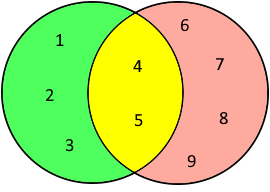# Symmetric Difference

Calculate the Symmetric Difference of two sets

## Calculate the Symmetric Difference

This function returns the symmetric difference of two sets. The symmetric difference of two sets is the set of elements that are in one of the sets but not in both.

To calculate, enter the two sequences of numbers. The individual numbers are separated by semicolons or spaces. Then click on the 'Calculate' button.

Symmetric Difference Calculator

 1st Set 2nd Set ResultThe symmetric difference is $$\{1, 2, 3, 6, 7, 8, 9\}$$

### Example of the symmetrical difference

The symmetric difference $$\displaystyle A \setminus B$$ consists of the elements contained in A or B but not in the intersection. It is comparable to the exclusive OR function in switching algebra.

The symmetric difference of $$\{1, 2, 3, 4, 5\}$$ and $$\{4, 5, 6, 7, 8, 9\}$$ is $$\{1,2, 3,6,7,8,9\}$$, because $$\{4,5\}$$ is contained in $$A$$ and $$B$$.